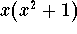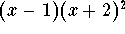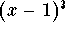#### Exercise 5.

Every quadratic polynomial has either 2 distinct real roots, one real root of multiplicity 2, or 2 complex roots. What cases can occur for a polynomial of degree 3? Give an example for each of these cases.

We know that there are 3 roots counted according to their multiplicity, and that complex roots show up in conjugate pairs. Thus, a little bit of puzzling reveals that there are four cases.

Case 1. Three real roots, each of multiplicity 1; an example is the polynomial (x-1)(x-2)(x-3).

Case 2. One real root, one complex conjugate pair; an example is the polynomial.

Case 3. Two real roots, one of multiplicity 1, the other one of multiplicity 2; an example is.

Case 4. One real root of multiplicity 3; an example is.[Back] [Exercises] [Next]
[Algebra] [Trigonometry] [Complex Variables]
[Calculus] [Differential Equations] [Matrix Algebra]S.O.S MATHematics home page

Do you need more help? Please post your question on our S.O.S. Mathematics CyberBoard.Helmut Knaust
Tue Jun 24 09:55:04 MDT 1997